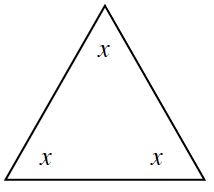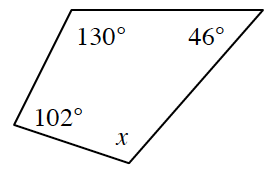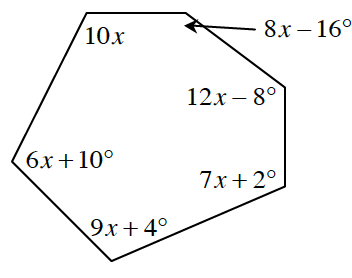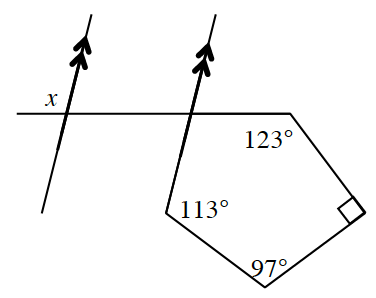### Home > INT2 > Chapter 8 > Lesson 8.4.2 > Problem8-94

8-94.

Solve for $x$ in each diagram below. Show your work.

Review the Math Notes box in Lesson 8.2.2 for help determining the sum of the interior angles of a polygon.

1.$x = 60^\circ$

1.$x = 82^\circ$

1.$10x + (8x - 16) + (12x - 8) +$ ... = ?

1.How are $∠x$ and the missing angle in the polygon related?